# Clock

How many hours are 15 days?

Result

n =  360 h

#### Solution:Leave us a comment of example and its solution (i.e. if it is still somewhat unclear...):

Showing 0 comments:Be the first to comment!## Next similar examples:

1. Hours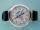How many hours is 9 days?
2. Time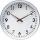16.2 days ..... how many hours is it?
3. HoursThe lesson lasts 45 minutes. For the week, students have 18 lesson hours. How many are the actual hours?
4. Nightingale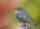Nightingale sang five seconds for 5 hours and 5 minutes. How many seconds were missing to sing 10 hours?
5. Water tankIn an empty fire tank, 2150 hl of water jetted in 5 hours. How many hectoliters of water was jetted every hour? How many hectoliters of water was in the tank after three hours?
6. Weeks38 weeks is equal to how many days?
7. Hr to minSue biked to school in 5/12 of an hour. How many minutes did it take her to ride to school?
8. TheatreTheatrical performance started at 15:50 and ended at 18:50. How many minutes long?
9. Pump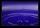From the pump in the yard dripping water. Every drop is 1 ml. How many drops will be necessary before filling a bucket with a volume of 10 l? Calculate how long it will fulfill the bucket when drops fall each second.
10. Father and son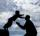Father is 44 years old, his son 16 years. Determine how many years ago was the father five times older than the son.
11. Fridays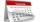Friday 13th is in 4 days. What day is today and what day it is?
12. Speeds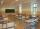George pass on the way to school distance 200 meters in 165 seconds. What is the average walking speed in m/s and km/h?
13. A trip to the peak Dry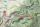Calculate what percentage is rising trip from Strečno to peak Suchý (Dry peak) longer than the descent back. Team trio of tourists (Palo, Peter and Andrew) rise took 4:21 and descent 3:08.
14. Minutes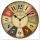Determine the difference in minutes: T1 = 2 3/20 h T2 = 2.3 h
15. ProductResult of the product of the numbers 1, 2, 3, 1, 2, 0 is:
16. TriangleProve whether you can construct a triangle ABC, if a=9 cm, b=10 cm, c=4 cm.
17. Roman numerals +Add up the number writtens in Roman numerals. Write the results as a decimal number.